Area Of Cone

Chapter 13 Class 9 Surface Areas and Volumes
Concept wise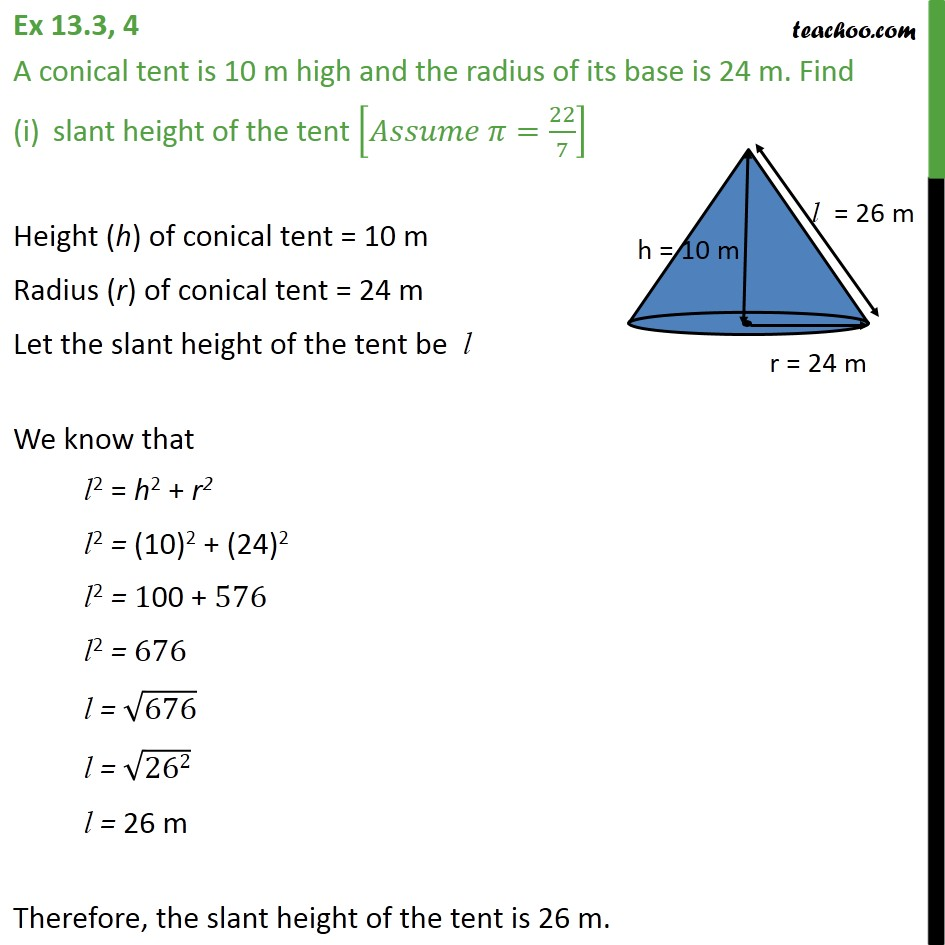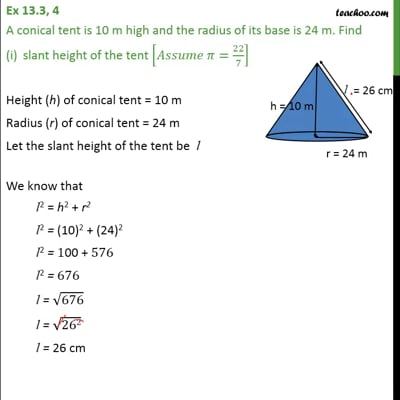This video is only available for Teachoo black users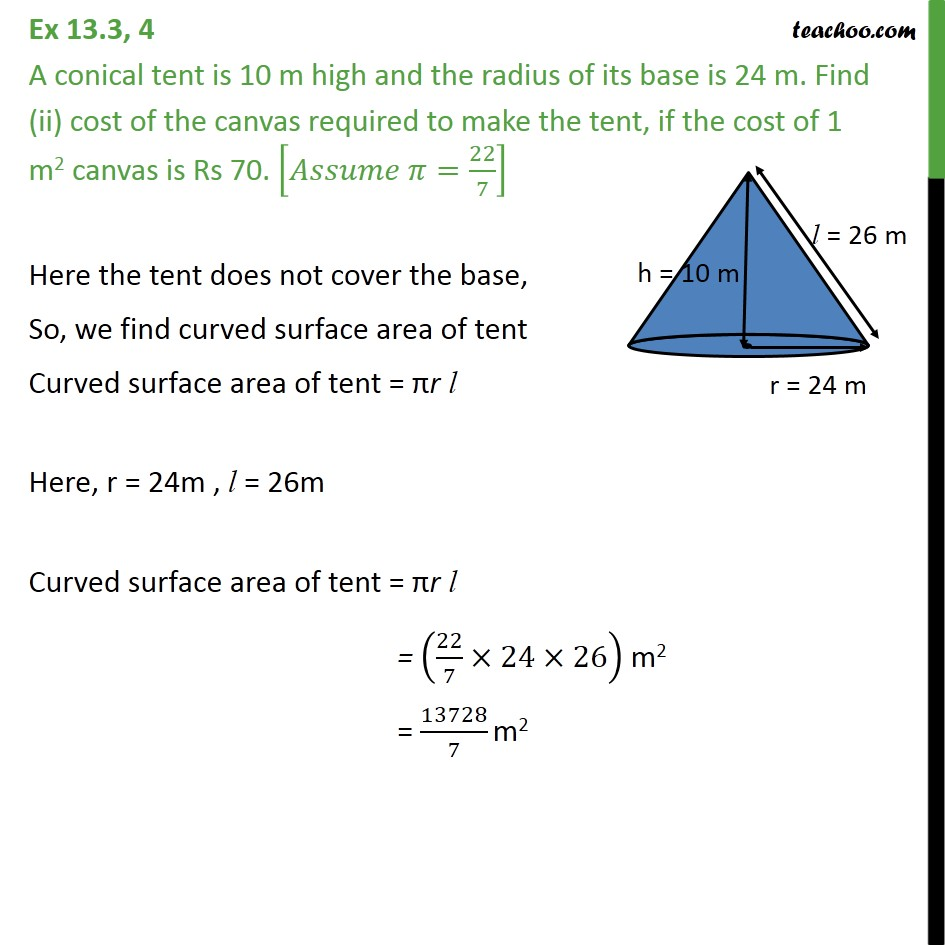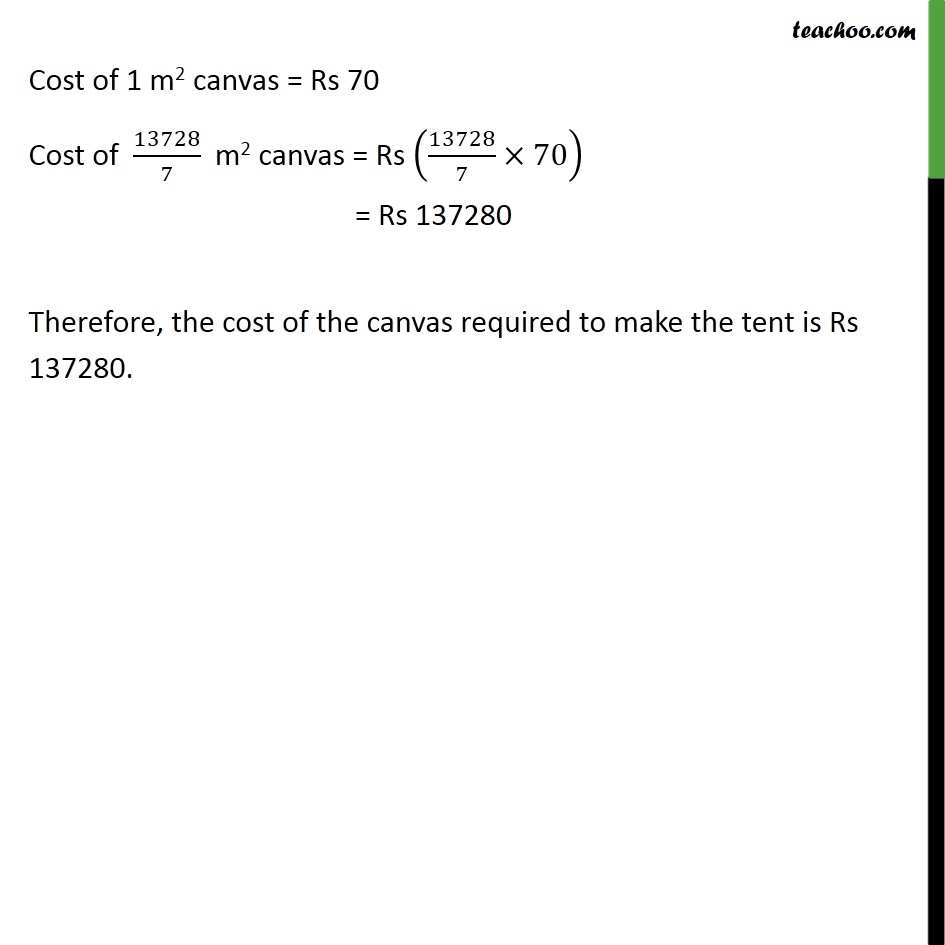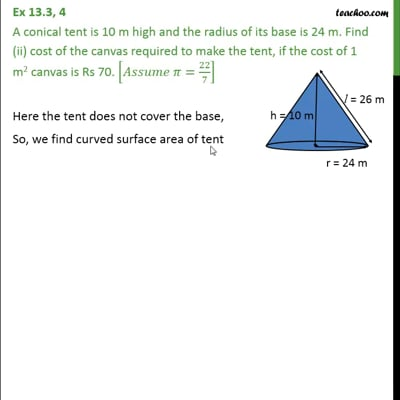This video is only available for Teachoo black users

Introducing your new favourite teacher - Teachoo Black, at only ₹83 per month

### Transcript

Ex 13.3, 4 A conical tent is 10 m high and the radius of its base is 24 m. Find slant height of the tent [𝐴𝑠𝑠𝑢𝑚𝑒 𝜋=22/7] Height (h) of conical tent = 10 m Radius (r) of conical tent = 24 m Let the slant height of the tent be l We know that l2 = h2 + r2 l2 = "(10)2 + (24)2" l2 = "100 + " 576 l2 = 676 l = √676 l = √("262" ) l = 26 m Therefore, the slant height of the tent is 26 m. Ex 13.3, 4 A conical tent is 10 m high and the radius of its base is 24 m. Find (ii) cost of the canvas required to make the tent, if the cost of 1 m2 canvas is Rs 70. [𝐴𝑠𝑠𝑢𝑚𝑒 𝜋=22/7] Here the tent does not cover the base, So, we find curved surface area of tent Curved surface area of tent = πr l Here, r = 24m , l = 26m Curved surface area of tent = πr l = (22/7×24×26) m2 = 13728/7 m2 Cost of 1 m2 canvas = Rs 70 Cost of 13728/7 m2 canvas = Rs (13728/7×70) = Rs 137280 Therefore, the cost of the canvas required to make the tent is Rs 137280.